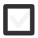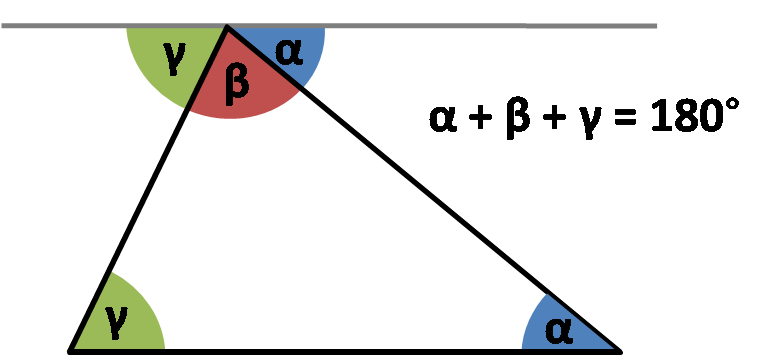# Use the angle sum in triangle. Draw rhombus, cone, pyramid, globe, parallelogram.383 views

## 2 lessons

+1 vote

First let's see a very nice way how to practically prove that triangles always have 180° sum of angles.
Check out this video:

Cool right? You can try this yourself with any triangle you care to cut out.
Try and see if you can make one whose angles don't add up to 180.Image source: Omnicalculator.com

This means that at all times we can calculate the third angle if we know the other two in any triangle.

So let's try this out!

EXERCISE:

Let's say we have a triangle just like one in the image above and let's say it's known angles are α=25°, β=75° (this doesn't necessarly have to be true for the image above, we use triangle images often more as a sketch than an actual drawing). The third angle, angle γ is unknown and we need to find it. Therefore we write:

α=25° β=75° γ=?

α + β + γ = 180°

γ = 180°- (α + β)

α + β = 25° + 75° = 100°

γ = 180°- 100°
γ = 80°

So we calculated the third angle by substracting the sum of first two from 180°.

+1 vote

DRAW A PARALELOGRAM OR RHOMBUS

In order to draw paralelogram or rhombus we should first discuss what kind of shape is a paralelogram or a rhombus.

Both paralelogram and rhombus are quadrilaterals, meaning they are 2 D geomtrical shapes with four sides, four vertices and four angles.

What is specific about paralelogram is that it has it opposite sides paralel and equal in length (but only the opposite ones not all four) and also opposite angles are equal while angles on the same side are suplemental (they add up to 180°).Image Sourced from http://www.moomoomath.com/parallelogram_rules.JPG

So to draw paralelogram we just have to have these properties in mind. Take a look at this video:

Also you can try and construct paralelogram with a ruler and a compass which can be even more fun. Check out this video:

As for rhombus we are going to do the same as rhombus is just a sort of special type of paralelogram whose all four sides are the same. So everything we said about paralelogram applies to rhombus only here all four sides are equal in length. As a result of that rhombus also has diagonalls that intersect under right angles.

You can draw a rhombus the same way you did paralelogram, just pay attention to equal sides. Here however is another intersting way with a ruler and a compass: